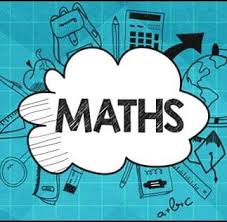# Odd and Even Numbers

## Worksheets

SUBJECT: MATHEMATICS
LEVEL: STANDARD ONE
STRAND: NUMBERS
TOPIC: ODD AND EVEN NUMBERS TO 100

By the end of this worksheet, you will be able to:

• Differentiate between odd and even numbers.

Key Points:

• An even number of objects can be divided into groups of two without leaving a remainder.

Example: Eight marbles can be divided into four groups of two groups without a remainder, as seen below:• An odd number of objects cannot be divided into groups of two without leaving a remainder. In other words, one is always left out of the group.

Example: Five marbles cannot be divided into groups of two without leaving one out, as seen below:• Even numbers end with the digits 0, 2, 4, 6, or 8.
Example: 20, 32, 44, 56, and 68 are all even numbers.
• Odd numbers end with the digits 1, 3, 5, 7, or 9.
Example: 21, 33, 45, 57 and 69 are all odd numbers.

Resources: Use the link below to enrich your understanding of even and odd numbers: